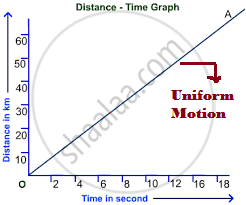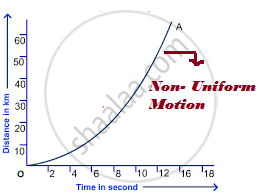Share

# Describing Motion - Uniform Motion and Nonuniform Motion

#### notes

Uniform Motion:A body is said to be in uniform motion if it travels equal distances in equal intervals of time, no matter how small these time intervals may be. If we draw distance time graph for uniform motion then it will be straight line. For better understanding we can take an example, a car is running at a constant speed say 20 metres per second, will cover equal distances of 20 metres, every second, so its motion will be uniform.

Non-Uniform Motion:A body is said to be in a non-uniform motion if it travels unequal distances in equal intervals of time. For example, if we drop a ball from the roof of a tall building, we will notice that it will cover unequal distances in equal intervals of time. Like, 5 metres in the 1st second, 15 metres in the 2nd second and so on. Thus, a freely falling ball will covers smaller distances in the first ‘1 second’ and larger distances in the later ‘1 second’ intervals. Therefore, we can say that the motion of a freely falling body is an example of a non-uniform motion. The distance-time graph of the non-uniform motion is a curved line. Non-Uniform motion is also known as accelerated motion.

 Basis of difference Uniform Motion Non-Uniform Motion Definition or meaning When a body moves along a straight line with uniform speed or steady speed is called Uniform motion When a body moves along a straight line but with variable or change in speed is called non-uniform motion How much distance is covered? Covers equal distance in equal intervals of time Covers unequal distances in equal intervals of time What is average speed here? Same as the actual speed of the object Different from actual speed of the object Rectilinear motion Acceleration is zero Acceleration is non-zero Distance-time graph Here distance-time graph shows straight line Here distance-time graph shows curved line

### Shaalaa.com

Motion part 5 (Uniform and non-uniform motion) [00:10:26]
S
series 1
0%

S